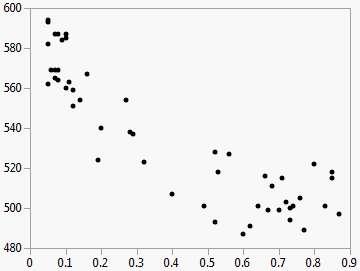Basic Analysis > Bivariate Analysis
Publication date: 11/29/2021

# Bivariate Analysis

##### Examine Relationships between Two Continuous Variables

Use the Bivariate platform to investigate the relationship between two continuous variables. Interactively add a simple linear regression line, polynomial regression curves, or smoothers to a scatter plot. Using the scatterplot, you can see at a glance the relationship between the two variables.

The Bivariate platform is the continuous by continuous personality of the Fit Y by X platform. The word bivariate simply means involving two variables instead of one (univariate) or many (multivariate).

Figure 5.1 Example of Bivariate Analysis